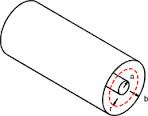# Foolish Electron in magnetic Field ...!!Two co-axial conducting cylindrical shells having radius 'a' and 'b' (such that b>a). A Uniform Magnetic field of strength 'B' is existing between cylindrical shells only which is directed along axis of cylinders.

An electron is emitted (ejected) by the inner shell in radially outward direction with velocity 'v'.

Find the MAXIMUM value of velocity 'v' so that this electron will not strike the outer cylindrical shell. ( Give answer in decimals upto 2 places )

Details and Assumptions:

1) Neglect all other forces except Magnetic forces.All values are in SI unit system.

2)$eB=9.1\times { 10 }^{ -31 }, a=5, b=10$,e=charge on electron.

3)${m}_{e}$=mass of electron = $9.1\times { 10 }^{ -31 }$